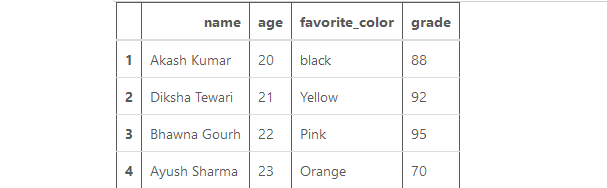Open in App
Not now

# Sort the Pandas DataFrame by two or more columns

• Last Updated : 17 Aug, 2020

In this article, our basic task is to sort the data frame based on two or more columns. For this, Dataframe.sort_values() method is used. This method sorts the data frame in Ascending or Descending order according to the columns passed inside the function.

First, Let’s Create a Dataframe:

## Python3

 `#import python library``import` `pandas as pd`` ` `# dictionary``data_frame ``=` `{``       ``'name'``: [``'Akash Kumar'``, ``'Diksha Tewari'``,``                ``'Bhawna Gourh'``, ``'Ayush Sharma'``],``        ``'age'``: [``20``, ``21``, ``22``, ``23``],``        ``'favorite_color'``: [``'black'``, ``'Yellow'``, ``                           ``'Pink'``, ``"Orange"``],``        ``'grade'``: [``88``, ``92``, ``95``, ``70``]``}`` ` `# create data frame with indexing``df ``=` `pd.DataFrame(data_frame, ``                  ``index ``=` `[``1``, ``2``, ``3``, ``4``])`` ` `# printing the dataframe``df`

Output:Example 1: Sort Dataframe based on ‘age'(in descending order) and ‘grade’ (in ascending order) column.

## Python3

 `# sort the dataframe``# based on age and grade``df.sort_values([``'age'``, ``'grade'``],``              ``ascending ``=` `[``False``, ``True``])`

Output:Example 2: Sort Dataframe based on ‘name’ and ‘favorite_color’ column in ascending order.

## Python3

 `# sort the dataframe based ``# on name and favorite_colr``df.sort_values([``'name'``, ``'favorite_color'``], ``               ``ascending``=``[``True``,``                          ``True``])`

Output:Example 3: In-place sorting of Dataframe based on ‘grade’ and ‘favorite_color’ column. In case of in-place sorting, Dataframe.sort_values() method returns nothing it performs changes in the actual dataframe.

## Python3

 `df.sort_values([``"grade"``, ``"favorite_color"``], ``               ``axis ``=` `0``, ascending ``=` `True``, ``               ``inplace ``=` `True``,``               ``na_position ``=``'first'``)`` ` `# printing the dataframe``df`

Output:My Personal Notes arrow_drop_up